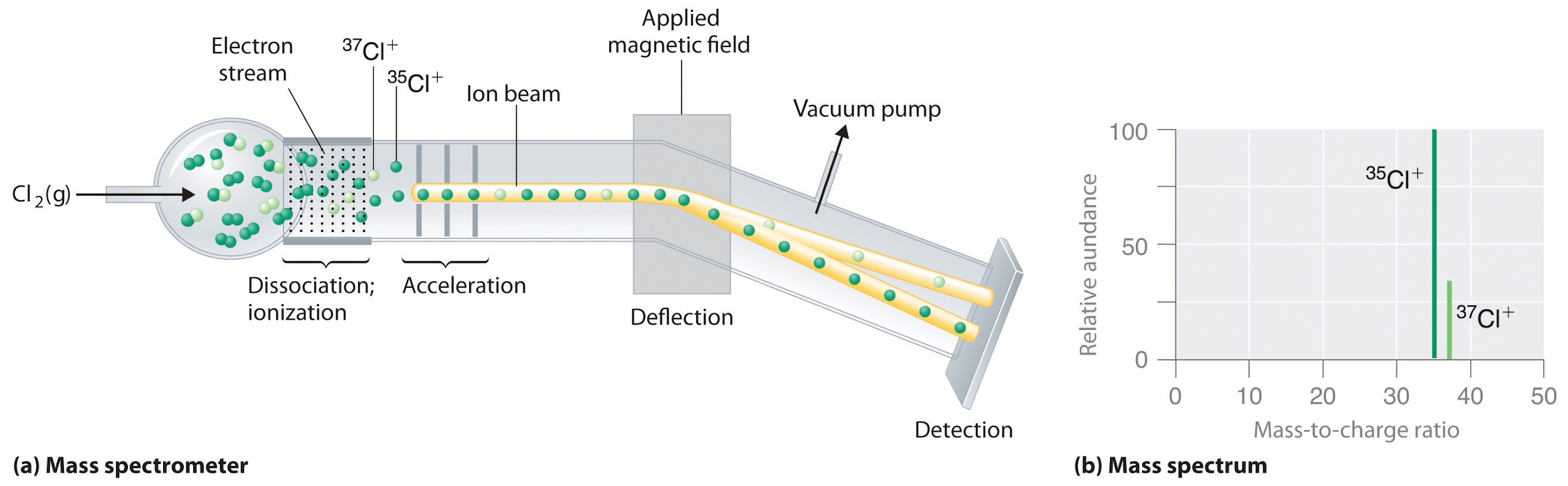The relative abundance of isotopes is the percentage of isotopes present in a sample of an element. By using mass spectrometry, the relative atomic masses are determined which are further used to calculate the percentage relative abundance.

Outline

## Relative abundance of Isotopes

The relative abundance of isotopes is the percent of one isotope occurring in nature.

Isotopes of a same atom differ in neutron number, as their proton number (atomic identity) remains same. Different isotopes present in different amounts within an element, so it is convenient to find relative abundance of each isotope.

For example, there are two isotopes of chlorine in nature which are 35Cl and 37Cl. To find the average atomic mass of an element, one must find the relative abundance of its isotopes. Mass spectrometer is used to determine relative atomic masses.

## Determination of relative atomic mass using mass spectrometer

Mass spectrometer allows the measurement of mass to charge ratio from which the relative mass of isotopes is determined. The vapourized sample of chlorine enters an ionizing chamber with high energy electrons. These electrons give enough energy to ionize the chlorine gas by taking off the electron. These ions are then passed through a magnetic field. Under a specific magnetic field, deflection of ions occurs depending upon masses (or speed) of ions. The heavier ion, moving at slow speed, deflected more than the ion which has lighter mass. This deflection recorded at the detector and the peaks shown on the mass spectrum depicts the relative abundance of isotopes.## Average atomic mass

From mass spectrometer data, the relative masses of atoms are obtained. This data is further used to calculate the average atomic mass of atoms of an element. The formula is as follow

For example average atomic mass for isotopes of chlorine 35Cl and 37Cl is:

## Percentage abundance

The formula for percentage isotopic abundance is given as:

M1(x) + M2(1 – x) = Me ……. (1)

• M1 = mass of isotope 1
• M2 = mass of isotope 2
• Me = average atomic mass of element given in periodic table

x = fraction of relative abundance of one isotope

(1 – x) = fraction of relative abundance of second isotope

To calculate the percentage abundance, one should follow the steps below:

1. Find average atomic mass of the element given in periodic table
2. After finding the values of mass of isotopes and element, determine the value of ‘x’ by solving above equation (1)
3. As the ‘x’ is a number so to get percentage abundance, multiply that number by 100.

For example:

The chlorine has two isotopes 35Cl and 37Cl. Equation (1) is used to determine their percentage abundance.

• M1 of 35Cl = 35 amu
• M2 of 37Cl = 37 amu
• Me = 35.50

35 (x) + 37 (1 – x) = 35.50

35x + 37 – 37x = 35.50

37 – 2x = 35.50

37 – 35.5 = 2x

x = 0.75

(1 – x) = 0.25

The value of x comes out 0.75 for 35Cl, so its percentage is 75%. On the other hand, (1 -x) for 37Cl comes out to be 0.25, so its percentage abundance is 25%.

Percentage abundance of 35Cl

## Concepts Berg

How do you calculate relative abundance

The relative abundance of isotope comes out by solving ‘x’ in below equation

M1(x) + M2(1 – x) = Me

M1 = mass of isotope 1

M2 = mass of isotope 2

Me = average atomic mass of element given in periodic table

x = fraction of relative abundance of one isotope

(1 – x) = fraction of relative abundance of second isotope

How to calculate percentage abundance using atomic and isotopic masses

The relative abundance of the isotopes can be find from equation

M1(x) + M2(1 – x) = Me

The ‘x’ obtained from this equation multiply by 100 to calculate the percentage abundance of isotopes.

What is the abundance of chlorine 35

In a sample of chlorine, Cl-35 is 75% abundant as compared to Cl-37 which is 25% present in that sample.

What is the relative abundance of isotopes?

The relative abundance of isotopes is the percentage of isotopes present in a sample of naturally occurring elements.

How do you find the relative abundance of two europium isotopes

Europium has two isotopes which are Eu-151 and Eu-153. The atomic mass of europium is 151.96. So, to find relative abundance using following process

151 (x) + 153 (1 – x) = 151.96

x = 0.52

By getting the value of x the relative abundance of Eu-151 and Eu-153 are determined which are 52% and 48% respectively.

What is the relative abundance of neon isotopes

Neon has three isotopes which are Ne-20, Ne-21, Ne-22. Ne-20 has 90.48% abundant in nature while Ne-21 and Ne-22 has 0.27% and 9.25% abundant respectively.

What is relative intensity and how is it related to percent abundance

The relative intensity is the intensity of line or peak in a spectrum. Its value is the same as percentage abundance for a given isotope.

References